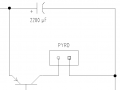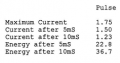# Calculating the Total Energy Released by a Capacitor up to Defined Points in Time

Thread Starter

#### TAS142

Joined Feb 21, 2018
1
Hi All,

First ever post here. I am not at all an electronics expert. In fact, I'm pretty useless, yet due to resource availability, this task has fallen on me.

Here is my situation. I currently have a device which, amongst other things, charges a capacitor such that on activation of a switch, the main power supply is removed and the capacitor kicks in which subsequently activates a pyrotechnic device used as part of a release mechanism.

Due to confidentiality reasons, I am not able to present the whole circuit diagram, but I have attached a modified snippet of the section of the circuit concerned. I'll be frank, I am not entirely sure of the function of the transistor, but I don't think this should influence my query too much. In terms of the pyro, in testing we have attached a 'dummy load' in the form of a resistor so I have been treating it as such. I guess for this purpose, the pyro can be viewed as a resistor.

Now, to get to my actual query. I have positioned a probe either side of the pyro/dummy load and connected to an oscilloscope which can give me a readout in terms of voltage or current. In both instances, on activation of the switches, the capacitor discharges and produces a discharge curve, as expected (a pretty typical looking exponential decay curve). What I am desperately trying to calculated is the total energy released up to certain time intervals (not that it impacts the process much, these time intervals are 5ms and 10ms). I have been told various things and despite using numerous attempts using various formulae and methodologies (lots of mentions of time constants, C=Q/V etc., E= I^2*R etc.), I have been unsuccessful. Historically we have used an automated programme produced by a subcontractor around 2 decades ago which did all the work for us once the device was plugged in. Unfortunately, this machine has now seen it's last days, so we need to find a manual method of it. The machine when it was working would produce a test certificate as a printout, which would present the required results. I have an extract of this certificate (attached) which provides currents and energies from which I have been trying to work backwards to try and figure out how they achieved the desired results. However, as I previously mentioned, I am failing terribly, so any guidance anyone can provide would be greatly appreciated!#### kubeek

Joined Sep 20, 2005
5,753
I am not sure what kind of oscilloscope you have, so there are two options. If you have a fancy one it can do math, and it should be able to multiply the current times voltage to get power, and then integrate that power over your 5 or 10ms to get the energy.
Not so fancy scope should be able to save the measured data, so you could for example save it to excel sheet and do the math there on a computer.

#### OBW0549

Joined Mar 2, 2015
3,566
The energy stored in a capacitor, in joules, is equal to one-half the capacitance, in farads, times the square of the voltage across it, in volts.

So if you know the capacitance and can measure the voltage across it with a scope, you can calculate the energy remaining in that capacitor at any given time; and the difference between the energy at the instant discharge begins, and the energy remaining at any point thereafter, gives you the energy delivered to the load.

#### AnalogKid

Joined Aug 1, 2013
9,262
The transistor is acting as an electronically-controlled on/off switch.

The total energy in a capacitor is:

E = 1/2 x C x V^2 -- Energy equals one-half times the capacitance (in farads) times the square of the voltage.

The unit is watt-seconds, abbreviated Ws, or W-s

To calculate a partial energy, calculate it at the starting voltage and at the ending voltage, and subtract. Neither voltage has to be "fully charged" or "empty".

ak

#### Janis59

Joined Aug 21, 2017
1,332
The energy W(c)=C*U^2/2 BUT if the capacitor is not fully charged-up, then U=e^-(t/tau) where tau=R*C, where in CC mode the R(equiv)=EDS/i(charge-up)